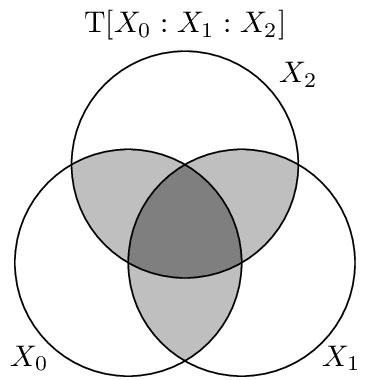# Total Correlation¶

The total correlation [Wat60], denoted $$\T{}$$, also known as the multi-information or integration, is one generalization of the Mutual Information. It is defined as the amount of information each individual variable carries above and beyond the joint entropy, e.g. the difference between the whole and the sum of its parts:

$\begin{split}\T{X_{0:n}} &= \sum \H{X_i} - \H{X_{0:n}} \\ &= \sum_{x_{0:n} \in X_{0:n}} p(x_{0:n}) \log_2 \frac{p(x_{0:n})}{\prod p(x_i)}\end{split}$

Two nice features of the total correlation are that it is non-negative and that it is zero if and only if the random variables $$X_{0:n}$$ are all independent. Some baseline behavior is good to note also. First its behavior when applied to “giant bit” distributions:

In : In : from dit import Distribution as D


So we see that for giant bit distributions, the total correlation is equal to one less than the number of variables. The second type of distribution to consider is general parity distributions:

In : In : from dit.example_dists import n_mod_m


Here we see that the total correlation is equal to $$\log_2{m}$$ regardless of $$n$$.

The total correlation follows a nice decomposition rule. Given two sets of (not necessarily independent) random variables, $$A$$ and $$B$$, the total correaltion of $$A \cup B$$ is:

$\T{A \cup B} = \T{A} + \T{B} + \I{A : B}$
In : In : from dit.multivariate import coinformation as I


## Visualization¶

The total correlation consists of all information that is shared among the variables, and weights each piece according to how many variables it is shared among.## API¶

total_correlation(dist, rvs=None, crvs=None, rv_mode=None)[source]

Computes the total correlation, also known as either the multi-information or the integration.

Parameters
• dist (Distribution) – The distribution from which the total correlation is calculated.

• rvs (list, None) – A list of lists. Each inner list specifies the indexes of the random variables used to calculate the total correlation. If None, then the total correlation is calculated over all random variables, which is equivalent to passing rvs=dist.rvs.

• crvs (list, None) – A single list of indexes specifying the random variables to condition on. If None, then no variables are conditioned on.

• rv_mode (str, None) – Specifies how to interpret rvs and crvs. Valid options are: {‘indices’, ‘names’}. If equal to ‘indices’, then the elements of crvs and rvs are interpreted as random variable indices. If equal to ‘names’, the the elements are interpreted as random variable names. If None, then the value of dist._rv_mode is consulted, which defaults to ‘indices’.

Returns

T – The total correlation.

Return type

float

Examples

>>> d = dit.example_dists.Xor()
>>> dit.multivariate.total_correlation(d)
1.0
>>> dit.multivariate.total_correlation(d, rvs=[, ])
0.0

Raises

ditException – Raised if dist is not a joint distribution or if rvs or crvs contain non-existant random variables.